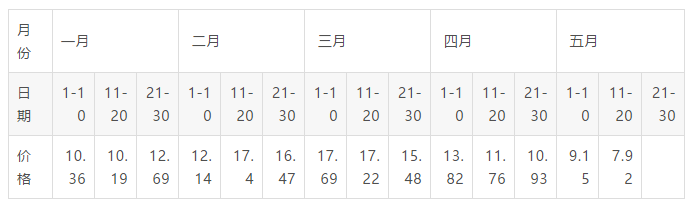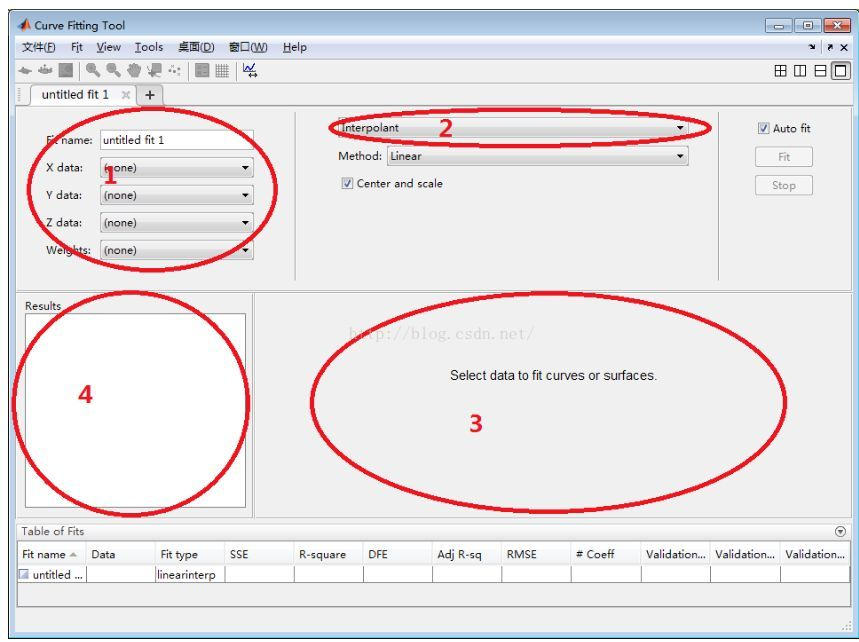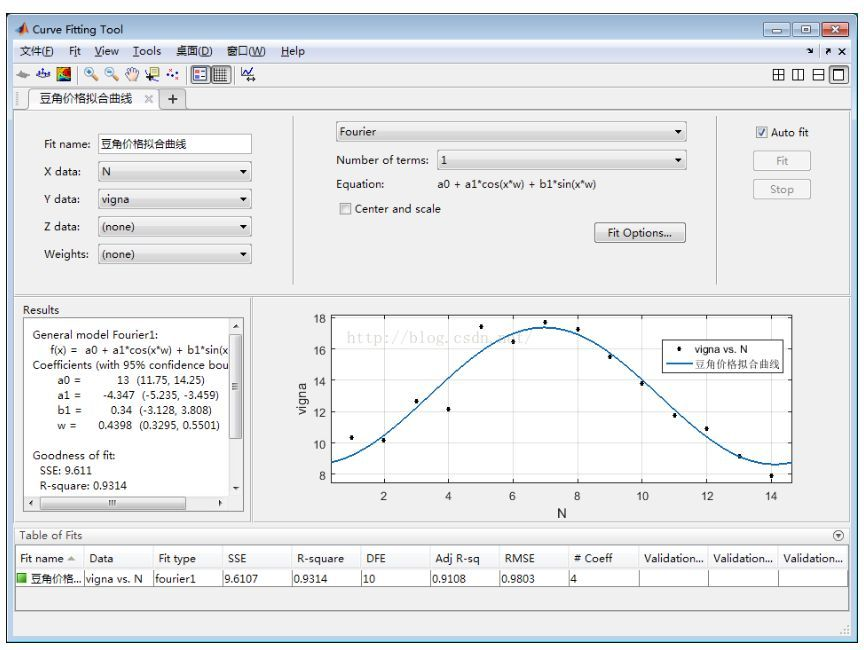• MATLAB数据拟合例子(一次函数、指数函数、双曲线)(2010-06-03 01:44:30)转载▼分类：数学工具标签：杂谈一次函数：(a+bx = y)%先求出拟合函数format long;x = [2001 2002 2003 2004 2005 2006 2007 2008 2009];y = ...

MATLAB数据拟合例子(一次函数、指数函数、双曲线)

(2010-06-03 01:44:30)转载▼

分类：数学工具

标签：杂

一次函数：(a+bx = y)

%先求出拟合函数

format long;

x = [2001 2002 2003 2004 2005 2006 2007 2008 2009];

y = [32.2 31.3 29.7 28.6 27.5 26.1 25.3 23.7 22.7];

d = [1 1 1 1 1 1 1 1 1];

a=[d;x];

b = a*y';

a=a*a';

c=a\b

c =

1.0e+003 *

2.436797222221444

-0.001201666666666

%所以，拟合函数为 y = 1.0e+003 *(2.436797222221444 - 0.001201666666666*x %根据拟合函数求估测值

format short;

x = [2010, 2011, 2012, 2013, 2014]

1.0e+003 *(

2.436797222221444 - 0.001201666666666*x)

ans =

21.4472 20.2456 19.0439 17.8422 16.6406

展开全文matlab拟合例子
• 函数拟合知道y=a+b*exp(-x)观测数据x0.0 0.3 0.8 1.1 1.6 2.3y0.820.72 0.63 0.60 0.55 0.5求a、b，线性回归或拟合问题。建立矛盾方程组AX=y其中X=[a,b]'这里将a、b看做待求量，X=A\y或由最小二乘准则X=inv(A'A)*A'...

函数拟合

知道y=a+b*exp(-x)

观测数据

x 0.0 0.3 0.8 1.1 1.6 2.3

y 0.82 0.72 0.63 0.60 0.55 0.5

求a、b，线性回归或拟合问题。

建立矛盾方程组

AX=y

其中X=[a,b]'

这里将a、b看做待求量，X=A\y或由最小二乘准则X=inv(A'A)*A'y

t=[ 0.0 0.3 0.8 1.1 1.6 2.3]'

y=[0.82 0.72 0.63 0.60 0.55 0.5]'

A=[ones(size(t)),exp(-t)]

x=inv(A'*A)*A'*y%x=A\y

n=500

tt=zeros(n,1);

yy=zeros(n,1);

dt=2.3/n;

for i=1:n

tt(i)=i*dt;

yy(i)=x(1)+x(2)*exp(-tt(i));

end

plot(t,y,'b',tt,yy,'r')X=A\y

得X=0.4760

0.3413

函数拟合为y=0.4760+0.3413*exp(-x)

展开全文• 原标题：Matlab数据拟合工具在数学建模中的简单应用01 问题描述下表是由中国国家统计局提供的《50个城市主要食品平均价格变动情况》整理得到的2016年1月到5月豆角价格数据表，请建立数学模型解决下来两个问题：(1)...

原标题：Matlab数据拟合工具在数学建模中的简单应用

01 问题描述

下表是由中国国家统计局提供的《50个城市主要食品平均价格变动情况》整理得到的2016年1月到5月豆角价格数据表，请建立数学模型解决下来两个问题：

(1)豆角价格有什么特点？

(2)对6月份豆角价格变化情况进行预测。02 模型假设，符号说明

2.1 模型假设

一个国家的宏观经济会呈现周期性，因此作为一国宏观经济的组成部分之一的物价水平也会呈现周期性，随着经济周期的上下波动，物价水平也会上下波动。基于这点，描述物价水平的数学模型——经验公式也应该具有周期性。我们不妨假设描述物价水平数学模型是一个周期函数，并且可以转化为傅里叶级数。

2.2 符号说明

从表中，我们不难发现，国家统计局会在一个月里对50个城市主要食品平均价格变动情况进行三次调查统计，分别是1-10日，11-20日和21-30日三个时间区间。因此，描述物价水平的周期函数的自变量是月份。为了方便处理，我们再做一次抽象处理，将时间抽象为整数N，其中0<=N<=36。那么如何使用N来表示某一个月呢？又如何使用N来表示某一个月里的第几次调查呢？为此，我们使用如下两个运算符(高级编程语言中都有这两个运算符)：

/：整除运算符，比如13/3=4

%:取模运算符，比如13%3=1

这样整数N与月份和当月的第几次调查之间的关系可以表示如下：

调查次序Q=N%3

如果Q=0，那么调查月份M=N/3，否则M=N/3 + 1

比如取N=13，那么调查次序Q=1，调查月份M=5，这表示5月份的第一次调查，即调查时间为5月1-10日。

03 数学建模

接下来看看，如何使用MATLAB的数据拟合工具解决该问题。

设时间矩阵N = [1 2 3 4 5 6 7 8 9 10 11 12 13 14]；

豆角价格的矩阵vigna= [10.36 10.19 12.69 12.14 17.4 16.47 17.69 17.22 15.48 13.82 11.76 10.93 9.15 7.92]；

3.1 进入数据拟合窗口

在MATLAB命令行窗口中输入cftool，回车即可。3.2 数据拟合

在区域1中选择相应坐标轴的变量名，在区域2中选择拟合的函数模型，在区域3会会自动显示拟合的图像，在区域4中会给出拟合后的函数表达式。

区域2中可以选择的函数模型有：

Custom Equation 自定义方程

Exponential 指数拟合

Fourier 傅里叶拟合

Gaussian 高斯拟合

Interpolant 插值

Linear Fitting 线性拟合

Polynoimal 多项式

Power 幂函数拟合

Rational 有理拟合，两个多项式之比，分子和分母都是多项式

Smoothing Spline 平滑样条

Sum of Sine 正弦曲线拟合

Weibull 韦布尔拟合

区域4中显示拟合结果的评价指标，含义如下：

SSE：Sum of Squares due to Error误差平方和，越接近0曲线的拟合效果(由最小二乘法计算得出)

R-square：越接近1，曲线的拟合效果越好

RMSE：root mean square error 均方根误差，越接近0曲线的拟合效果

最后看看本题的拟合效果，如下图所示。最后看看，区域4中显示的函数模型：

General model Fourier1:

f(x) = a0 + a1*cos(x*w) + b1*sin(x*w)

Coefficients (with 95% confidence bounds):

a0 = 13 (11.75, 14.25)

a1 = -4.347 (-5.235, -3.459)

b1 = 0.34 (-3.128, 3.808)

w = 0.4398 (0.3295, 0.5501)

Goodness of fit:

SSE: 9.611

R-square: 0.9314

RMSE: 0.9803

通过各种函数模型的拟合发现这个模型拟合效果最好，R-square: 0.9314，非常接近于1。

因此描述豆角价格变化规律的数学模型就是：

vigna = f(N) = 13 + -4.347*cos(N*0.4398) + 0.34*sin(N*0.4398)

这是一个周期函数，符合经济周期的规律。

至此，本题基本解决了。

04 总结

本文通过一个数学建模题目讲解了MATLAB中数据拟合工具的使用，该工具比较简单，但是功能很强大。MATLAB中含有许多功能强大的工具，读者有兴趣的话，可以继续探索，在使用该工具时，也可以从软件设计的角度去欣赏，个人觉得MATLAB软件的设计是一个经典的学习例子。

End.

责任编辑：

展开全文• 3.1 生成数据xxdata为2 的x数据。 function dydt=modelsq(t,y,k,v) dydt =k(1)*(y-6).^k(2)+v; clear; k=[-3.548 3.236]; y0=6; x=[0 1 2 3 4 5 7 9 11 14 17 20 25 30 35 40 45 53 60 70 80 90 100 110 120 130 ...

3变参数微分方程系数回归

您好斑竹，我的最终目的是要识别k参数，我的微分方程模型为

y'=k(1)(y-y0)*y^k(2)+v ;

(我的参数识别方法是参考一个例子，后面给贴出来。但是这个例子是不带v输入变量的)

1 我前面一段程序先假设k=3,然后利用变参量数值解法求解了(仿真)y值；(因为这个式子有个带外部输入的参数v，没法求得解析解，所以只好用数值微分方程方法求出ydata；

2 我后面一段程序就是利用前边得到的t值和y值去识别参数k；

3.1 生成数据xxdata为2 的x数据。

function dydt=modelsq(t,y,k,v)

dydt =k(1)*(y-6).^k(2)+v;

clear;

k=[-3.548 3.236];

y0=6;

x=[0 1 2 3 4 5 7 9 11 14 17 20 25 30 35 40 45 53 60 70 80 90 100  110 120 130 140 150]';%给出x值

fraction=500;%计算出500个数据；

t1=min(x):(max(x)-min(x))/fraction:max(x);

v1=1:length(x);

v=spline(x,v1,t1);

yy=y0;

for i=1:length(t1)-1

[t y]=ode23s(@modelsq,[t1(i) t1(i+1)],y0,[],k,v(i));

y0=y(end,: );

yy=[yy;y0];

end

xydata=[t1',yy,v'];

save('xydata.txt','xydata','-ascii');%把tt和yy存为txt文件。

3.2 参数识别

方法1 lsqcurvefit

function dydt=modelsq(t,y,k,v)

dydt =k(1)*(y-6).^k(2)+v;

function yi=num_value(k,x)

global v;

y0=6;

yy=y0;

for i=1:length(x)-1

[t y]=ode23s(@modelsq,[x(i) x(i+1)],y0,[] ,k,v(i));

y0=y(end,: );

yy=[yy;y0];

end

yi=yy;

clear;

global  v;

xdata=xydata(:,1);

ydata=xydata(:,2);

v=xydata(:,3);

k0=[4000,2000]'; %初值也可以改为k0=[-6,2]'

options=optimset('TolFun',1e-8,'TolX',1e-8,'MaxFunEvals',300, 'Algorithm','trust-region-reflective', 'display', 'iter');

lb=[-13 1];ub=[-1 5];

kp=lsqcurvefit(@num_value,k0,xdata,ydata,lb,ub,options);

%kp =[-3.5400,3.23000];

方法2 multistart和lsqnonglin, 全局算法(速度比较慢)

global  v;

xdata=xydata(:,1);

ydata=xydata(:,2);

v=xydata(:,3);

F =@(k)num_value(k,xdata)-ydata;

ms=MultiStart('Display','iter', 'UseParallel','always');

matlabpool open 2;

opts=optimset('Algorithm','trust-region-reflective');

problem=createOptimProblem('lsqnonlin','x0',[-4,2],'objective',F,'lb', [-6 1],'ub',[-1 5],'options',opts);

[xminm,fminm,flagm,outptm,manyminsm]=run(ms,problem,100)

matlabpool close

opts=optimset('Algorithm','trust-region-reflective','MaxTime',200，'Maxiter',400);

方法3 lsqcurvefit 和multistart

global  v;

xdata=xydata(:,1);

ydata=xydata(:,2);

v=xydata(:,3);

ms=MultiStart('Display','iter','UseParallel','always');

opts=optimset('Algorithm','trust-region-reflective');

matlabpool open

problem=createOptimProblem('lsqcurvefit','x0',[-4,2], 'objective',@num_value,'xdata',xdata,'ydata',ydata,'lb',[-6,1],'ub',[-1,5],'options',opts);

[xminm,fminm,flagm,outptm,manyminsm]=run(ms,problem,100)

方法4 globalsearch

global  v;

xdata=xydata(:,1);

ydata=xydata(:,2);

v=xydata(:,3);

F=@(k)norm(num_value(k,xdata)-ydata);

gs=GlobalSearch('Display','iter');

matlabpool open

opts=optimset('Algorithm','interior-point', 'UseParallel','always');

problem=createOptimProblem('fmincon','x0',[-4,2],'objective',F,'lb',[-6 1],'ub',[-1 5],'options',opts);

[xming,fming,flagg,outptg,manyminsg] = run(gs,problem)

方法5 模拟退火

tic;

global  v;

xdata=xydata(:,1);

ydata=xydata(:,2);

v=xydata(:,3);

F=@(k)norm(num_value(k,xdata)-ydata);

X0 =[-4,2];% Starting point

lb=[-6 1];ub=[-1 5];

options= saoptimset('Display','iter');

[x,fval,exitFlag,output] = simulannealbnd(F,X0,lb,ub,options)

time=toc;

你也要做类似的东西吗，我已经根据bainhome提示修改正确的程序，已发表到百度文库http://wenku.baidu.com/view /b4bcbf41be1e650e52ea99c5.html，http://wenku.baidu.com/view /ac184d25ccbff121dd3683c5.html，这两篇文章都是我上传的。

[本帖最后由 weiniuzhu 于 2011-2-28 22:13 编辑]

展开全文• 课程设计名称： 设计二：数据拟合 指导教师： 张莉 课程设计时数： 6 课程设计设备：安装了Matlab 、C ++软件的计算机 课程设计日期： 实验地点： 第五教学楼北902 课程设计目的：1. 了解最小二乘拟合的原理，掌握用...
• MATLAB数据拟合实例(给出两组数据拟合y=ax±b)转-MATLAB插值与拟合(1)2010-03-29 19:45§1曲线拟合实例：温度曲线问题气象部门观测到一天某些时刻的温度变化数据为：t012345678910T1315171416192624262729试描绘出...
• matlab数据拟合

2021-08-25 14:58:26
数据拟合 clc,clear; x=[0.5,1.0,1.5,2.0,2.5,3.0]; y=[1.75,2.45,3.81,4.80,7.00,8.60]; p=polyfit(x,y,2); x1=0.5:0.05:3.0; y1=polyval(p,x1); plot(x,y,'*r',x1,y1,'-b'); result p=polyfit(x,y,n)：最小...matlab
• 龙源期刊网 http://www.doczj.com/doc/2f22f716cbaedd3383c4bb4cf7ec4afe05a1b148.html基于matlab曲线拟合数据...这样给数据的分析和预测带来了极大的麻烦，本文针对股票数据的变化，使用matlab的多项式拟合，求...
• MATLAB数据拟合

2021-05-01 21:52:44
MATLAB 数据拟合 准备数据 准备原始数据，写入csv文件。 MATLAB载入数据 data = csvread('dataset.csv', R1, C1) % 读取数据，help csvread获取csvread函数用法 data_x = data[:, 1] % 获取自变量 data_y =...
• 两种方法 1.工具箱使用步骤（更详细）...2. matlab代码 %自定义拟合函数f(t)=a*cos(k*t)*exp(w*t) clc,clear syms t x=[0;0.4;1.2;2;2.8;3.6;4.4;5.2;6;7.2;8;9.2;10.4;11.6;12.4;13.6;14.4;15];%列向量 x1 = [0.042;0.
• MATLAB快速拟合二组数据 第一步：打开MATLAB，点击主页中的新建变量，点击修改变量名为a，然后复制数据进去，接着新建变量b，复制数据进去。 第二步：点击上端的APP，选择第一个图标 第三步：选择X轴变量和Y轴变量...vhdl fpga matlab
• 本来主要介绍机器学习、曲线拟合中常见的损失函数MSE的定义以及它的求导特性。 数理统计中均方误差是指参数估计值与参数值之差平方的期望值，记为MSE。MSE是衡量“平均误差”的一种较方便的方法，MSE可以评价...matlab MSE SSE RMSE
• 1.问题描述下表是由中国国家统计局提供的《50个城市主要食品平均价格变动情况》整理得到的2016年1月到5月豆角价格数据表，请建立数学模型解决下来两个问题：(1)豆角价格有什么特点？(2)对6月份豆角价格变化情况进行...
• 一次函数：(a+bx = y)%先求出拟合函数format long;x = [2001 2002 2003 20042005 2006 2007 2008 2009];y = [32.2 31.329.7 28.6 27.5 26.1 25.3 23.7 22.7];d = [1 1 1 1 1 1 1 11];a=[d;x];b = a*y';a=a*a';c=abc ...
• 想把这组数据拟合成一种适合的分布，第一组类似正态分布，第二组和第三组应该是偏态分布 希望得到：1.每组数据有一个函数表达式； 2.参数值； 3.误差分析。 理论上应该是： y1=f（x） y2=f(x*cos60°) y3=f(x*cos30...
• 使用matlab的Curve Fit Tool 进行数据拟合，选择多项式模式，从3阶到4阶切换时，对验证数据的测试相对误差偏大，而且大的离谱 原因： 拟合系数的默认显示位数为4 解决方式： 1）选择拟合界面上的 save to … 2）...matlab 数据处理
• 答：首先，你需要去除该Excel中的文字、字母部分，把它变成纯数据的表格，假设该表格文件名为“data.xls”，则在MATLAB程序中可编写如下： a = load('data.xls'); X = a(:,1); Y = a(:,2); Z = a(:,3); 以上即能完成...
• 篇一 : matlab获取等高线的数据contour(X,Y,Z,v)画出Z在向量v所有值处的等高线，如只想画出Z在i处的等高线，则调用contour(X,Y,Z,[i,i])。如果没有图形，可以将contour3试试看。另外，若想获得某一等高线处的具体...
• [matlab 曲线拟合(含实例)]matlab 曲线拟合 - 非常好非常全面的介绍M 拟合的参考资料Mathworks Tech-Note 1508 曲线拟合向导1． 介绍2． Mathworks 产品的曲线拟合特色a． 曲线拟合工具箱 (Curve Fitting Toolbox)b...
• 两组数据，用cftool拟合后 发现拟合出来的图不能编辑，也不能把sse r-sqeuar 表明在图上，这样的图paper里不能用，所以我想从新画一张图，既有散点图，又有cftool拟合出的幂函数和指数函数光滑曲线，所以还请大家给...
• Matlab指定拟合表达式的拟合

千次阅读 2021-01-07 22:04:27
Matlab的simplify函数化简符号表达式 此处只讨论用法，函数分析日后补充 用法：对一个符号式子同类项的系数进行合并同时按照幂次从高到底进行排列。必须得是一个符号式子，否则没有意义 如下代码： ...matlab
• 怎样用matlab拟合出形如 y = c1 + c2*to + c3*tc + c4*to^2 + c5*to*tc + c6*tc^2 + c7*to^3 + c8*tc*to^2 + c9*to*tc^2 + c10*tc^3的公式，即计算出此10项式的系数[c1,c2,c3,c4,c5,c6,c7,c8,c9,c10] 哪一个大神能...
• 借助MATLAB(R2017b)分布拟合工具箱distributionFitter，我们可以快速地检查数据的分布。 可以进行非参数密度估计(核平滑方法)和参数密度估计(Beta分布、gamma分布、Normal分布等十几个常用的参数模型)。给出参数估计...
• 一次函数：(a+bx = y)%先求出拟合函数format long;x = [2001 2002 2003 20042005 2006 2007 2008 2009];y = [32.2 31.329.7 28.6 27.5 26.1 25.3 23.7 22.7];d = [1 1 1 1 1 1 1 11];a=[d;x];b = a*y';a=a*a';c=a\bc...
• matlab拟合函数

2021-04-19 02:50:35
曲线拟合的例题1、 关于y=f(x)有一些观测数据x=-2*pi:0.1*pi:2*pi,时相应的函数值为y =[ 0.0000 0.8726 1.3803 1.3876 1.0490 0.6667 0.46120.4365 0.4293 0.2848 -0.0000 -0.2848 -0.4293 -0.4365-0.4612 -0.6667 -...
• ycalc = fh(x,nonLinMdl.Coefficients.Estimate('p1'),nonLinMdl.Coefficients.Estimate('p2')) plot(x,yval_,x,ycalc) 我无法上传文件，因为我在受限制的环境中，但我绘制了两个系列，模型计算值非常接近输入数据 ...
• 摘要：MATLAB下有三个数据拟合命令，即nonlinfit、lsqnonlin以及lsqcurvefit。这三个数据拟合命令是在最小二乘的基础上用于解决一些非线性方程的拟合优化问题。本文将从三个命令各自的依托算法、调用方法、调用结果......

matlab数据拟合matlab 订阅